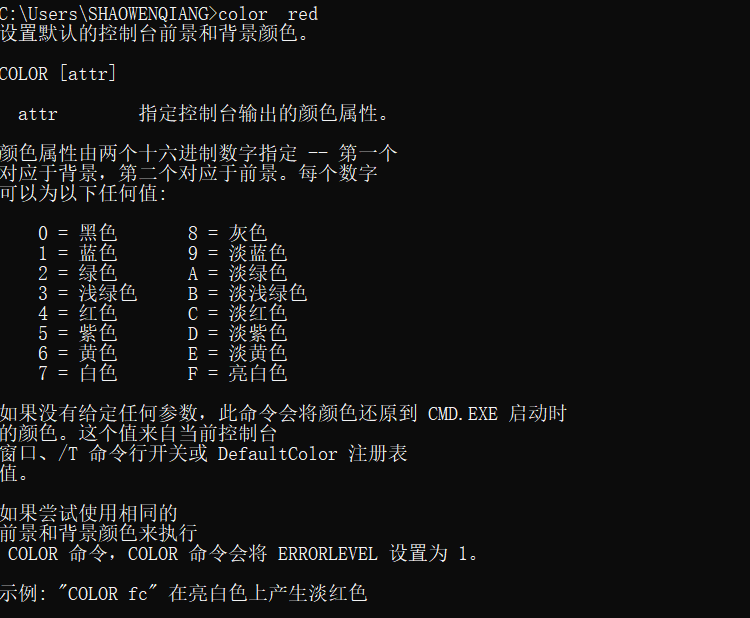# Windows 改变CMD窗口颜色！COLOR [attr]

attr 指定控制台输出的颜色属性。

0 = 黑色       8 = 灰色
1 = 蓝色       9 = 淡蓝色
2 = 绿色       A = 淡绿色
3 = 浅绿色     B = 淡浅绿色
4 = 红色       C = 淡红色
5 = 紫色       D = 淡紫色
6 = 黄色       E = 淡黄色
7 = 白色       F = 亮白色


COLOR 命令，COLOR 命令会将 ERRORLEVEL 设置为 1。